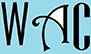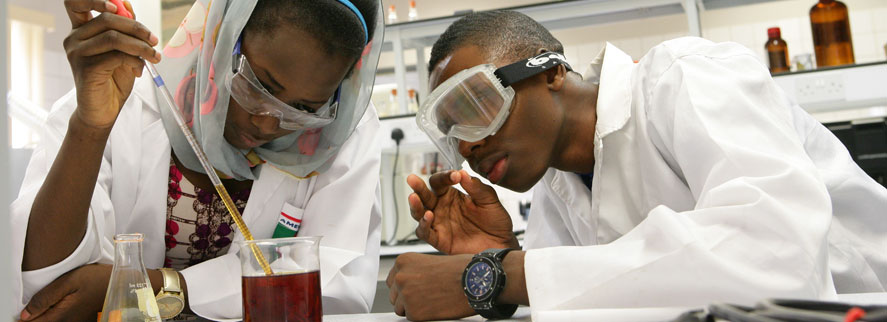## Geometry Question for Title 1 Schools: Circle Angle Question?

4) What is the value of an angle of the rest of the circle that has an angle of 90o?

The total angle of a circle = 360o

If part of the total angle is already 90o ,  then the rest must be 360o  – 90o

= 270º